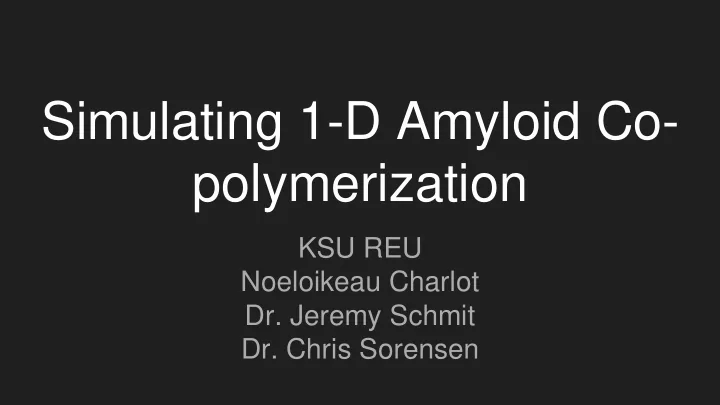# Simulating 1-D Amyloid Co- polymerization KSU REU Noeloikeau - PowerPoint PPT Presentation

## Simulating 1-D Amyloid Co- polymerization KSU REU Noeloikeau Charlot Dr. Jeremy Schmit Dr. Chris Sorensen Introduction Amyloid : polypeptides which polymerize to form cross-beta structures Co-polymer : a polymer consisting of two or

1. Simulating 1-D Amyloid Co- polymerization KSU REU Noeloikeau Charlot Dr. Jeremy Schmit Dr. Chris Sorensen

2. Introduction ● Amyloid : polypeptides which polymerize to form cross-beta structures ● Co-polymer : a polymer consisting of two or more monomers (subunits) The objective of this project is to simulate a one-dimensional amyloid copolymer consisting of two subunits: IAPP (amylin) and Aβ (amyloid beta). IAPP is secreted alongside insulin and has been linked to type-II diabetes. Aβ is the main component of amyloid plaques associated with Alzheimer’s. This project builds on work done by Sheena Radford (2017) on IAPP/Aβ amyloid copolymerization, and explores the case in which binding between dissimilar subunits is stronger than binding between similar subunits.

3. 2 Molecules, 3 Possible Chains and Reactions ● Two molecules, A & B ● Form linear chain ABAABABBABBAABAAAB… ● Can form Alternating, Block, or Random sequence ● Type depends on interaction energies: E ij ● Let I denote the last position in the chain ● Possible reactions: …I→...IA, …I→...IB, …I→..(I -1)

4. Algorithm Randomizes Reactions and Timesteps ● Let K 1 be reaction rate for addition of A ● Let K 2 be reaction rate for addition of B ● Let K 3 ∝ exp(-E ij ) be reaction rate for last element removal ● Let K tot = K 1 +K 2 +K 3 1. Generate random number: 0<R 1 <1 2. If: { R 1 ≤K 1 /K tot : add A to chain ; R 1 ≤(K 1 +K 2 )/K tot : add B ; else remove } 3. Generate random number: 0<R 2 <1 4. Update timestep defined by: t+= -ln(1-R 2 )/K tot

5. Parameter Space Consists of Concentration C, Binding Energy E, and Composition of Solution P ● Define order parameter: M = ( L ) -1 ∑ s i from i=1 to i=L where ● s = {-1: A ; +1: B } and L = length of chain M can be thought of as the composition of the aggregate polymer, and we observe changes in M by varying: ● Concentration: C ● Binding Energy: E ● Ratio of A to B: P P can also be thought of as the composition of the solution.

6. E=1: Composition of Aggregate Determined by Composition of Solution High Concentrations: ● M ≈ P Low Concentrations: ● M ≈ P ⇒ Linear.

7. E=1: Level Sets of P Observed in (M,C) Plane P=0.9 P=0.1

8. E=5: Composition of Aggregate Determined by Binding Energy at Low Concentrations High Concentrations ● M ≈ P Low Concentrations ● M ≈ constant ⇒ Sigmoidal.

9. E=5: Level Sets of P Observed in (M,C) Plane P=0.9 P=0.1

10. Recap: Composition of Aggregate is a Function of Energy and Concentration Small E : ● Composition of aggregate determined by composition of solution. ● Independent of concentration. Large E : ● Composition of aggregate depends on composition of solution only at high concentrations. ● At low concentrations, it is determined by the binding energy. Now let’s look at growth rate “ ”...

11. E=1: Growth Rate Independent of P ● Symmetric case: indistinguishable particles ● G ~ constant ● Different curves of P indistinguishable

12. E=2: Growth Rate Largest Closest to P=0.5 P= 0.6, 0.4 P= 0.5 P= 0.9, 0.1 ● Symmetry broken ● G ~ P ● Values of P closest to 0.5 show highest growth rate ● Chain alternates between A and B, maximizing number of strong bonds and minimizing number of off events

13. E=5: Growth Rate Begins at Lower Concentration P= 0.6, 0.4 P= 0.5 P= 0.9, 0.1 ● High asymmetry ● As E increases, G becomes nonzero at lower values of C ● This is because, at low concentrations, the stronger binding energy allows bonds between different types to persist in the time between addition events ● Hence we would expect the value of M to be zero in this region, which is precisely what was observed earlier

14. Growth Starts at Lower Concentrations for Higher Energies

15. Summary & Future Research ● At high concentrations, chain constituency determined by concentration. ● At low concentrations, chain constituency determined by binding energy. ● Chains with high binding energies begin growing at lower concentrations. ● Future research could account for different masses, charge, and structure.

More recommend